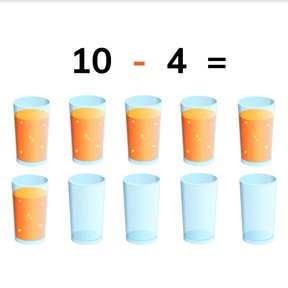Subtraction from 10 with numbers to 10

# Subtraction from 10 with numbers to 108,000 schools use Gynzy92,000 teachers use Gynzy1,600,000 students use Gynzy

## General

Students learn to subtract numbers to 10 from the number 10.

1.OA.A
1.OA.C.5
I.OA.C.6

## Relevance

Discuss with students that it is important to know how to subtract, so you can determine how many you have left when something is taken away. If you have 10 glasses of juice and 4 are emptied, you know that you have 6 full glasses left.

## Introduction

Set 10 pencil sharpeners on the table and ask a student come to the front. Have the student divide the pencil sharpeners into two groups. The class must then say which groups the student made with the pencil sharpeners. Repeat this with ten books and pens asking different students to come to the front to split the ten into two groups.

## Development

Hold 10 colored pencils. Take 1 pencil away and demonstrate that you now have 9 pencils left. Then again, hold 10 colored pencils. Take 2 away and demonstrate that you now have 8 pencils left. Start again with 10 colored pencils. Give 3 colored pencils to a student. Ask the class how many colored pencils you have now. Tell the class that this is 10 minus 3. You now have 7 pencils. You can continue demonstrating subtraction from 10 with the colored pencils or move to the interactive whiteboard. The interactive whiteboard shows an orange tree with 10 oranges. There are 10 images in which different amounts of oranges have fallen off of the tree. Discuss these with the students and show the accompanying subtraction problem. There are 10 oranges on the tree so every subtraction problem starts with 10.
Next show subtraction problems with balloons and cans. Remind students that you are starting with 10 and the second number is being taken away.
Discuss with students that the first number (minuend) tells how many objects there are, while the second number (subtrahend) tells how many are taken away. Take the second number from the first and you get your difference, or how many are left. Students are then asked to solve some subtraction problems from 10. Ask students to write their answers on a sheet of paper, and then to hold the paper up so you can check their work. Students can also use blocks or manipulatives in sets of 10 to tangibly take away the second number (subtrahend) from the original 10. Next explain to students that you can also get these subtraction problems without visual support that you can count. You then are only given the numbers. Discuss mental math strategies for counting in your head or imagining the blocks/objects. Do some exercises together as a class.

Check that students are able to subtract numbers to 10 from 10 by asking the following questions:
- How do you subtract?
- How do you solve the subtraction problem 10 - 5 = ...?

## Guided practice

Students first practice subtraction problems from 10 with visual support. They are then given the subtraction problems without visual support.

## Closing

Discuss with students that it is important to be able to subtract from 10, so you can determine how much you have left. Close with a subtraction coloring game. Students must solve the given subtraction problems and then color the matching squares in the grid. If all answers are correct, you should end up with a beautiful flower.

## Teaching tips

Students who have difficulty with subtraction can make use of manipulatives, like MAB blocks. Have them set out 10 blocks and then take away a given number and count how many are left.

## Instruction materials

pencil sharpeners, books, pens, colored pencils
optional: MAB blocks or other manipulatives

### The online teaching platform for interactive whiteboards and displays in schools

• Save time building lessons

• Manage the classroom more efficiently

• Increase student engagement